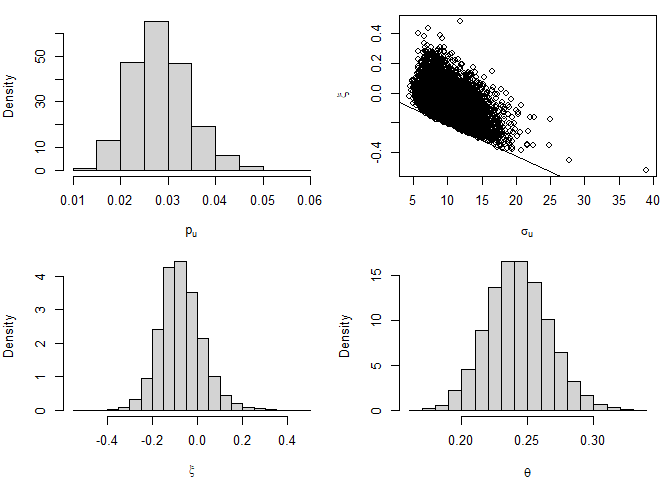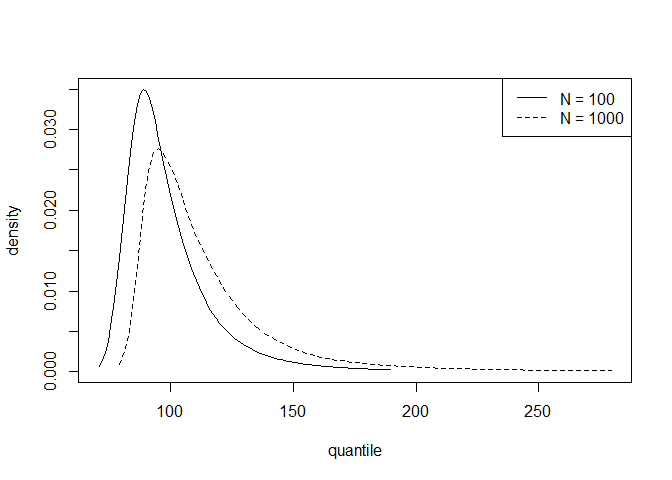# Bayesian Likelihood-Based Inference for Time Series Extremes

#### 2023-01-27

For information about the model underlying the inferences performed in this vignette, including the (adjusted) likelihood for $$(p_u, \sigma_u, \xi, \theta)$$ and the Cheeseboro wind gusts dataset used below, see the vignette Frequentist Likelihood-Based Inference for Time Series Extremes.

## Bayesian inferences for model parameters

We perform Bayesian inference for $$(p_u, \sigma_u, \xi, \theta)$$, combining a likelihood with a prior distribution for these parameters. For $$\theta$$ the likelihood is based on the $$K$$-gaps model. For $$(p_u, \sigma_u, \xi)$$ the likelihood is based on vertically-adjusted log-likelihoods for $$p_u$$ and $$(\sigma_u, \xi)$$. This latter aspect is an example of Bayesian inference using a composite likelihood (Ribatet, Cooley, and Davison (2012)).

Currently, lite allows only priors where $$p_u$$, $$(\sigma_u, \xi$$) and $$\theta$$ are independent a priori. In the example below, we use the blite function’s default priors for the exceedance probability $$p_u$$ (the Jeffreys’ prior), the generalised Pareto parameters $$(\sigma_u, \xi)$$ (and maximal data information prior) and $$\theta$$ (a uniform prior on [0,1]). Different priors can be set using the respective arguments gp_prior, b_prior and theta_prior_pars.

The blite function samples from the posterior distribution for $$(p_u, \sigma_u, \xi)$$ based on vertically-adjusted log-likelihoods for $$p_u$$ and $$(\sigma_u, \xi)$$. To use unadjusted log-likelihoods set the argument type = "none".

library(lite)
cdata <- exdex::cheeseboro
# Each column of the matrix cdata corresponds to data from a different year
# blite() sets cluster automatically to correspond to column (year)
cpost <- blite(cdata, u = 45, k = 3, ny = 31 * 24, n = 10000)

The summary and plot methods produce numerical and graphical marginal summaries of the posterior samples.

summary(cpost)
#>
#> Call:
#> blite(data = cdata, u = 45, k = 3, ny = 31 * 24, n = 10000)
#>
#>          Posterior mean Posterior SD
#> p[u]            0.02838     0.006032
#> sigma[u]        9.95900     2.376000
#> xi             -0.07280     0.094370
#> theta           0.24270     0.023430
plot(cpost)## Predictive inference

We perform posterior predictive inference for the largest value $$M_N$$ to be observed over a future time period of length $$N$$ years. Objects returned from the blite function have a predict method based on the predict.evpost method in the revdbayes package (Northrop 2020). See the Posterior Predictive Extreme Value Inference using the revdbayes Package vignette for information. The effect of adjusting for the values of the extremal index in the posterior sample is to change the effective time horizon from $$N$$ to $$\theta N$$.

The function predict.blite can estimate predictive intervals, quantiles, distribution and density functions for $$M_N$$ and simulate from the predictive distribution for $$M_N$$. For example, the following code estimates a 95% highest predictive density (HPD) interval for $$M_{100}$$ and plots the predictive densities of $$M_{100}$$ and $$M_{1000}$$.

# Interval estimation
predict(cpost, hpd = TRUE)\$short
#>         lower    upper n_years level
#> [1,] 73.01946 140.6364     100    95
# Density function
plot(predict(cpost, type = "d", n_years = c(100, 1000)))